### Home > CALC > Chapter 1 > Lesson 1.4.2 > Problem1-160

1-160.
1. The function y = x3 + 1 is graphed below, along with four left endpoint rectangles which approximate the area from x = −2 to x = 2. Homework Help ✎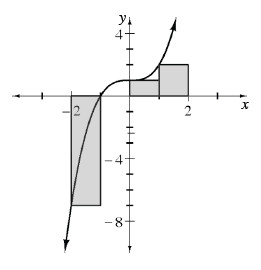1. Why does it look like there are only three rectangles?

2. Recall that area under the x-axis is negative, while that above the x-axis is positive. Approximate A(y, −2 ≤ x ≤ 2) using these four rectangles.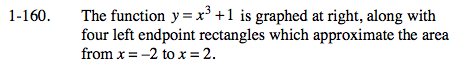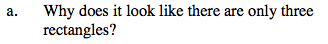What happens if the left endpoint is on the x-axis?

Since f(−1) = 0, the height of the rectangle is 0.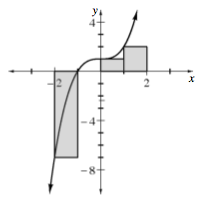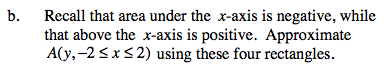Sum the areas of all four rectangles together. Remember to treat a rectangle's area as negative if it is below the x-axis.